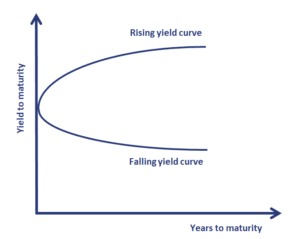# Theories of the Term Structure of Interest Rates

We discuss 5 different theories of the term structure of interest rates. Each of the different theories of the term structure has certain implications for the shape of the yield curve as well as the interpretation of forward rates. The five theories are the unbiased expectations theory, the local expectations theory, the liquidity preference theory, the segmented markets theory, and the preferred habitat theory.## Unbiased Expectations theory

The unbiased expectations theory or pure expectations theory argues that it is investors’ expectations of future interest rates that determine the shape of the interest rate term structure.  Under this theory, forward rates are determined solely by expected future spot rates. This means that long-term interest rates are an unbiased predictor of future expected short-term rates.

An important implication of the pure expectations theory is that an investor will earn the same return over a certain period, regardless of the bonds he or she purchases. Thus, buying a 3-year bond an holding to maturity will earn the same as buying a 1-year bond and investing the proceeds after one year in a 2-year bond.

The implications for the term structure of interest rates are as follows:

• if the curve is upward sloping, short-term rates are expected to rise
• if the curve is downward sloping, short-term rates are expected to fall
• a flat curve indicates that short-term rates are expected to remain unchanged

## Local expectations theory

The second theory is the local expectations theory. This theory is similar to the previous theory. There is is only one important difference. Risk-neutrality only holds for short holding periods. Over longer periods, risk premiums may exist.

The local expectations theory implies that over short holding periods, all investors will earn the risk-free rate. Empirical evidence rejects the local expectations theory. Even the short-holding-period returns for long-maturity bonds are higher than the short-holding-period returns for short-maturity bonds because of liquidity considerations.

## The liquidity preference theory

The liquidity preference theory tries to address one of the shortcomings of the pure expectations theory. The theory argues that forward rates also reflect a liquidity premium to compensate investors for exposure to interest rate risk. This liquidity premium is said to be positively related to maturity.

An important implication of the liquidity preference theory is the fact that forward rates are expected to be biased because the market’s expectation of future rates includes a liquidity premium. A positively sloping yield curve may thus be the result of expectation that short-term rates will go up or simply because of a positive liquidity premium. The size of the liquidity premium may also be time-varying.

## The segmented market theory

The segmented market theory argues that the term structure is not determined by either liquidity or expected spot rates. Instead, the shape of the yield curve is solely determined by the preference of borrowers and lenders. The yield curve at any maturity simply depends on the supply and demand for loans at that maturity. The yield at each maturity is independent of the yields at other maturities.

## Preferred habitat theory

The final theory is the preferred habitat theory. This theory argues that forward rates represent expected future spot rates plus a premium. The preferred habitat theory argues that, if there are imbalances between supply and demand at a particular maturity, then investors are willing to shift habitat in exchange for a premium. Here too, supply and demand dictate interest rates. The only difference with the segmented market theory is that lenders and borrowers are willing to change maturity in exchange for a premium.

## Summary

we discussed the 5 theories of the term structure of interest rates. While each of the theories has its merits, there is no consensus on which best explains the observed term structure.